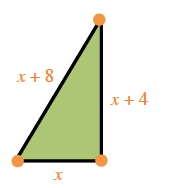### Home > PC > Chapter 6 > Lesson 6.4.2 > Problem6-158

6-158.

The lengths of the sides of a right triangle are given by $x$, $x + 4$, and $x + 8$. What are the lengths of the sides?

Draw a diagram to visualize.Since it is a right triangle, use the Pythagorean Theorem.